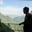Related Tags

r
histogram
vector
communitycreator

# What are histograms in R?AKASH BAJWA

A histogram shows the frequencies of values of a variable bucketed into ranges. A histogram is alike to a bar chart but the deviation is it groups the values into continuous ranges. Every bar in the histogram represents the height of the number of values present in that range.

R generates histograms using the hist() method. This function uses a vector as an input and takes some further parameters (for additional attributes) to plot histograms.

### Syntax

The basic syntax to generate a histogram using R is as follows:

hist(v, main, xlab, xlim, ylim, breaks, col, border)


### Parameters

• v: Vector that contains arithmetical values used in the histogram.
• main: Indicates the title of a chart.
• col: Taken to set the color of chat bars.
• xlab: Used to give brief information of x-axis.
• xab: Used to give a brief detail of x-axis.
• xlim: Used to define the range of values on the x-axis.
• ylim: Taken to define the range of values on the y-axis.
• Breaks: Used to show the width of each bar.
• border: Used to set the border color of all bars.

### Simple histogram plot

A simple histogram is generated by using the input vector, label, col, and border parameters. The information given below is provided to create and save the histogram in the current R working directory.

# First create data for graph.
v <- c(9,13,21,8,36,22,12,41,31,33,19)

# Make histogram
hist(v,
xlab = "weight",
col = "yellow",
border = "blue")

### Bins and breaks

To change the bin width you can specify Breaks as an argument including the breakpoints you want to have. You can do this by using c() method: Vector c(100,200,300,500,700) is being specified.

hist(x, breaks=c(100,200, 300, 500, 700))


### Range of x and y values

To set out the range of values allowed in the X-axis and Y-axis, we take the xlim and ylim parameters. The width of all bars can be selected by using breaks.

# Generate data for the graph
v<- c(9,13,21,8,36,22,12,41,31,33,19)

# Make histogram.
hist(v,
xlab = "Weight",
col = "green",
border = "red",
xlim = c(4,6),
ylim = c(1,2),
breaks = 5)

### Expected output

RELATED TAGS

r
histogram
vector
communitycreator

CONTRIBUTORAKASH BAJWA
RELATED COURSES

View all Courses

Keep Exploring

Learn in-demand tech skills in half the time